### 需要导入的包

import tensorflow as tf          # TensorFlow
import matplotlib.pyplot as plt  # matplotlib绘图
import numpy as np               # Numpy
from sklearn.metrics import confusion_matrix    # 混淆矩阵，分析模型误差

# notebook使用
%matplotlib inline


### 载入数据

TensorFlow在样例教程中已经做了下载并导入MNIST数字手写体识别数据集的实现，可以直接使用。以下代码会将MNIST数据集下载到data/MNIST目录下，将标签保存为one-hot编码。

from tensorflow.examples.tutorials.mnist import input_data


MNIST数据集总共有70000张手写数字图片，数据集被分为训练集、测试集和验证集三部分。

print("数据集大小：")
print('- 训练集：{}'.format(len(data.train.labels)))
print('- 测试集：{}'.format(len(data.test.labels)))
print('- 验证集：{}'.format(len(data.validation.labels)))

数据集大小：
- 训练集：55000
- 测试集：10000
- 验证集：5000


#### One-hot编码

print(data.test.labels[:5])

[[ 0.  0.  0.  0.  0.  0.  0.  1.  0.  0.]
[ 0.  0.  1.  0.  0.  0.  0.  0.  0.  0.]
[ 0.  1.  0.  0.  0.  0.  0.  0.  0.  0.]
[ 1.  0.  0.  0.  0.  0.  0.  0.  0.  0.]
[ 0.  0.  0.  0.  1.  0.  0.  0.  0.  0.]]


one-hot编码中，只有对应类别的那个位置为1，其余都为0，我们可以使用以下代码将其转换为真实类别：

data.test.cls = np.argmax(data.test.labels, axis=1)
print(data.test.cls[:5])

[7 2 1 0 4]


#### 数据维度

print("样本维度：", data.train.images.shape)
print("标签维度：", data.train.labels.shape)

样本维度： (55000, 784)



img_size = 28                        # 图片的高度和宽度
img_size_flat = img_size * img_size  # 展平为向量的尺寸
img_shape = (img_size, img_size)     # 图片的二维尺寸

num_classes = 10                     # 类别数目


#### 打印部分样例图片

def plot_images(images, cls_true, cls_pred=None):
"""
绘制图像，输出真实标签与预测标签
images:   图像（9张）
cls_true: 真实类别
cls_pred: 预测类别
"""
assert len(images) == len(cls_true) == 9   # 保证存在9张图片

fig, axes = plt.subplots(3, 3)   # 创建3x3个子图的画布

for i, ax in enumerate(axes.flat):
# 绘图，将一维向量变为二维矩阵，黑白二值图像使用 binary
ax.imshow(images[i].reshape(img_shape), cmap='binary')

if cls_pred is None:  # 如果未传入预测类别
xlabel = "True: {0}".format(cls_true[i])
else:
xlabel = "True: {0}, Pred: {1}".format(cls_true[i], cls_pred[i])
ax.set_xlabel(xlabel)

# 删除坐标信息
ax.set_xticks([])
ax.set_yticks([])

# 随机取9张图片
indices = np.arange(len(data.test.cls))
np.random.shuffle(indices)
indices = indices[:9]

images = data.test.images[indices]
cls_true = data.test.cls[indices]

plot_images(images, cls_true)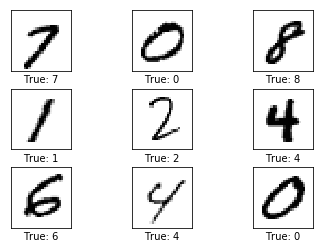### TensorFlow计算图

TensorFlow使用计算图模型来构建神经网络。其主要流程是先建立好整个网络的计算图模型，然后再导入数据进行计算。

• Placeholder: 占位符，用来读取用户输入与输出；
• Variable: 模型的变量，也称为参数，在计算过程中逐步优化；
• Model: 使用的神经网络模型，也可以使用一些简单的计算；
• Cost Function： 代价函数，也称损失函数，如何计算模型的误差；
• Optimizer： 优化器，使用哪种优化策略来降低损失。

#### Placeholder占位符

x = tf.placeholder(tf.float32, shape=[None, img_size_flat])
y_true = tf.placeholder(tf.float32, shape=[None, num_classes])
y_true_cls = tf.placeholder(tf.int64, shape=[None])


#### Variable变量

$$y = Wx+b$$

weights = tf.Variable(tf.zeros([img_size_flat, num_classes]))
biases = tf.Variable(tf.zeros([num_classes]))


#### Model模型

logits = tf.matmul(x, weights) + biases


tf.matmul表示矩阵乘法。上式返回的结果是一个 [None, num_classes] 的矩阵。logits是TensorFlow常用术语，这里不去考虑。这个结果离真实的分类还有一定的距离，我们需要使用一个softmax来对其归一化，以使得它的和为1。Softmax函数类似于计算了每一个维度的概率，其中最大的那个概率即对应它的类别。

y_pred = tf.nn.softmax(logits)
y_pred_cls = tf.argmax(y_pred, axis=1)


y_pred_cls 的计算与之前使用Numpy计算类别的方式相似，说明TensorFlow的许多操作都和Numpy是相通的。不同的是Numpy的计算是实时的，而TensorFlow只有在运行计算图时才会返回结果。

#### Cost Function代价函数

cross_entropy = tf.nn.softmax_cross_entropy_with_logits(logits=logits, labels=y_true)
cost = tf.reduce_mean(cross_entropy)    # 交叉熵平均值即代价


#### Optimization优化

optimizer = tf.train.GradientDescentOptimizer(learning_rate=0.5).minimize(cost)


#### 性能度量

correct_prediction = tf.equal(y_pred_cls, y_true_cls)  # 判断相等的元素
accuracy = tf.reduce_mean(tf.cast(correct_prediction, tf.float32))  # 计算准确率


### 运行TensorFlow计算图

#### 创建Session以及变量初始化

TensorFlow计算图运行在一个session中，计算之前需要先创建这个session，并初始化其中的一些变量（w 和 b），TensorFlow使用session.run()来运行计算图。

session = tf.Session()   # 创建session
session.run(tf.global_variables_initializer())   # 变量初始化


#### 执行优化的帮助函数

batch_size = 100   # 每一轮的数据量
def optimize(num_iterations):
for i in range(num_iterations):   # 迭代轮数
# TensorFlow自己实现了取MNIST下一个批次的操作，这里直接使用，不必深究
x_batch, y_true_batch = data.train.next_batch(batch_size)

# 把这批数据放在要喂入模型的dict里面
feed_dict_train = {x: x_batch, y_true: y_true_batch}

# 运行优化器，喂入数据
session.run(optimizer, feed_dict=feed_dict_train)


#### 评估性能的帮助函数

feed_dict_test = {x: data.test.images,
y_true: data.test.labels,
y_true_cls: data.test.cls}


def print_accuracy():
# 运行accuracy来计算acc
acc = session.run(accuracy, feed_dict=feed_dict_test)
print("测试集准确率: {0:.1%}".format(acc))   # 保留1位小数


def print_confusion_matrix():
cls_true = data.test.cls   # 真实类别
# 运行y_pred_cls计算出的真实类别
cls_pred = session.run(y_pred_cls, feed_dict=feed_dict_test)

# 使用scikit-learn的confusion_matrix来计算混淆矩阵
cm = confusion_matrix(y_true=cls_true, y_pred=cls_pred)

# 打印混淆矩阵
print(cm)

# 将混淆矩阵输出为图像
plt.imshow(cm, interpolation='nearest', cmap=plt.cm.Blues)

# 调整图像
plt.tight_layout()
plt.colorbar()
tick_marks = np.arange(num_classes)
plt.xticks(tick_marks, range(num_classes))
plt.yticks(tick_marks, range(num_classes))
plt.xlabel('Predicted')
plt.ylabel('True')


def plot_example_errors():
# 运行计算图，得到争取情况和预测结果
correct, cls_pred = session.run([correct_prediction, y_pred_cls],
feed_dict=feed_dict_test)
# 计算错误情况
incorrect = (correct == False)
images = data.test.images[incorrect]
cls_pred = cls_pred[incorrect]
cls_true = data.test.cls[incorrect]

# 随机挑选9个
indices = np.arange(len(images))
np.random.shuffle(indices)
indices = indices[:9]

plot_images(images[indices], cls_true[indices], cls_pred[indices])


#### 优化前的性能

print_accuracy()


测试集准确率: 9.8%


plot_example_errors()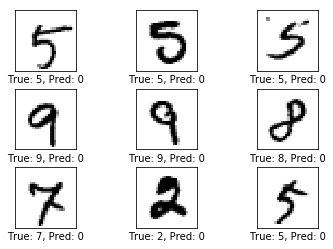#### 一轮迭代后的性能

optimize(num_iterations=1)
print_accuracy()

测试集准确率: 26.7%


plot_example_errors()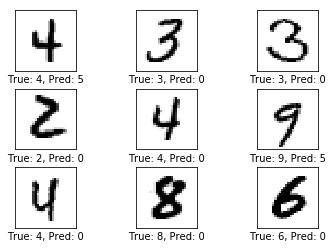plot_weights()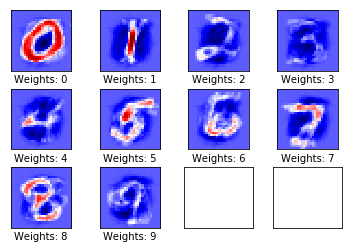#### 10轮迭代后的性能

optimize(num_iterations=9)   # 之前已经做过一轮
print_accuracy()

测试集准确率: 81.0%


plot_example_errors()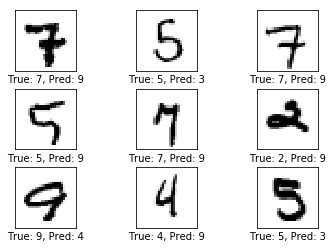plot_weights()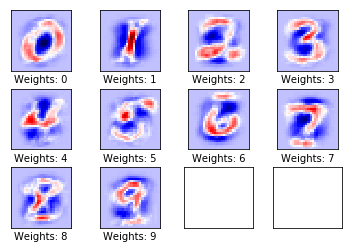#### 100轮迭代后的性能

optimize(num_iterations=990)   # 之前已经做过10轮
print_accuracy()

测试集准确率: 91.9%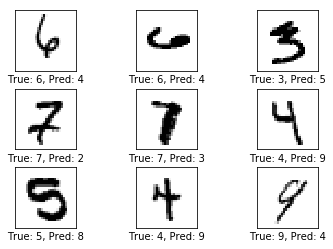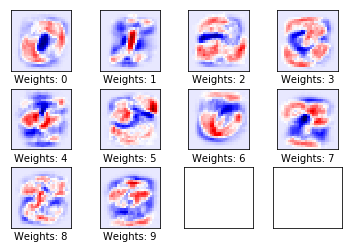print_confusion_matrix()

[[ 957    0    1    2    0    4   12    2    2    0]
[   0 1108    2    2    0    3    4    1   15    0]
[   6    7  922   20    5    3   19   11   32    7]
[   3    0   16  938    0   21    4    9   13    6]
[   2    1    6    3  897    1   22    2   10   38]
[   9    3    6   50    7  762   19    5   24    7]
[   9    3    3    2    6   12  921    1    1    0]
[   2   12   24   10    6    2    0  933    2   37]
[   7    6    7   38    9   32   16    8  844    7]
[  10    6    1   13   34    8    1   14    9  913]]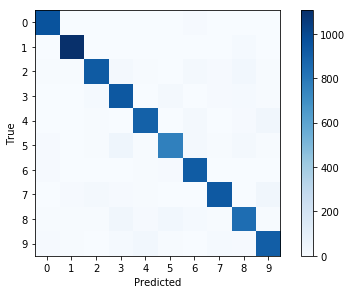session.close()